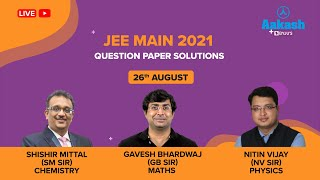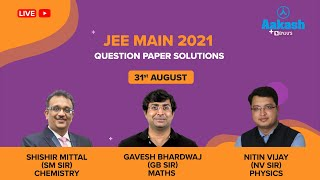# JEE Main 2021- Paper Analysis

In order to give candidates more time and chances, the JEE Main 2021 had four sessions, with two more sessions added to JEE Main. Thus, the candidates will have the following advantages.

• If a candidate is not able to do their best in the first attempt, they will be given 3 more chances to enhance their exam marks.
• Students will gain first-hand experience taking an exam on their first try, and they will learn from their mistakes so they may do better on their second try.
• This will lower the chance of the candidate losing a year.
• Any person who missed the exam due to unavoidable circumstances need not wait for a full year to retake the examination.
• A candidate need not appear for all four sessions. But, if the candidates appear in more than one session, the best score will be considered to prepare the merit list.

JEE Main Paper-1 included a total of 90 questions and the overall mark was 300. The question paper had three sections which included questions from maths, physics and chemistry. The marks allotted for each section are given below

 Total Questions 30 Questions Total Marks 100 marks Section 1 20 MCQs Section 2 10 Numerical Based Questions (Attempt any 5 questions) MCQs Marking Scheme +4 for correct answers -1 for incorrect answers Numerical Questions Marking Scheme +4 for correct answers 0 in all other cases

## JEE Main 2021 – Session 4 Day 1 Shift 2 – Analysis

• The questions in chemistry were all theory-based and mostly taken from the NCERT textbook.
• The maths session was quite long.
• The level of difficulty in physics was moderate.

## JEE Main 2021 – Session 4 Day 2 Shift 1 – Analysis

The overall difficulty level of the paper was moderate, according to the initial feedback from students who took the shift 1 exam.

Mathematics

Due to the lengthy calculations, the mathematics paper was considered simple, but time-consuming. Seven problems from calculus, five from coordinate geometry, four from vectors and three-dimensional, and at least five from algebra were asked. Nearly every topic was covered from maths.

Physics

As mentioned by the students, the physics section was the most challenging. There were 5 to 6 questions from electromagnetics, 8 from mechanics, 2 from units and dimensions, and 4 from optics. A few questions were included from other chapters.

## JEE Main 2021 – Session 4 Day 3 Shift 1 – Analysis

JEE Main 2021 – Session 4 Day 3 was conducted on August 31, 2021. Up to 1 lakh candidates appeared for the Joint Entrance Examination (JEE) during the two slots.

At least two numerical problems, one in physics and the other in chemistry, have been identified by students as being unsolvable. Some students have noted that the questions derived from the photoelectric effect were unclear. Regarding mathematics, a few students claimed that the paper was a little simpler than they had anticipated.

The expert analysis of the question paper is as follows

Mathematics

The mathematics portion was easy for a majority of students. As expected, some of the questions required extensive calculations. There were 7 to 8 calculus problems, at least 5 algebra questions, 3 to 4 questions about vectors and 3D, and 4 questions about coordinate geometry.

Physics

Physics paper was similarly of moderate difficulty level. Questions were asked from all the main chapters – 2 questions from optics, not less than 5 questions from mechanics, 2 or 3 questions from Thermodynamics and 2 questions from Semiconductors among others. As may be predicted, questions of a numerical nature focused more on numbers than on theory, and nearly all of them were based on straightforward formulas.

Chemistry

The Chemistry session was NCERT-based and easy to moderate. There were an equal number of questions from physical, inorganic, and organic chemistry. The majority of the questions in the segment with numerical values came from physical chemistry. The entire paper was based on NCERT. In addition to the usual topics, questions from biomolecules, polymers, and everyday chemistry were given fair coverage in the paper.

## JEE Main 2021 – Session 4 Day 4 Shift 2 – Analysis

The hardest of the three subjects was maths, whereas chemistry was the easiest. According to the students, this paper was overall of a moderate level.

Mathematics

The difficulty level of the examination was moderate to hard. Calculus, 3D, and Vector Algebra chapters received maximum weightage. Binomial Theorem, Permutation, Combination, Probability & Matrices, Properties of Definite Integral, and Differential Equation all had some excellent and challenging questions. Topics like Circle and Ellipse also had a few challenging questions.

Physics

The physics question was easy to moderate. The physics question paper was well balanced with practically every chapter being covered. There were some excellent questions from Kinematics, Electromagnetic Waves, Electromagnetic Induction, Heat & Thermodynamics, Semiconductors, Modern Physics, and Optics. Despite having long calculations, several questions with a numerical basis were simple.

Chemistry

Chemistry questions were the simplest. More emphasis was placed on organic and inorganic chemistry. Only numerical-based questions were asked from the Physical Chemistry chapter. A few questions were asked from General Organic Chemistry and Oxygen Containing Compounds in Organic Chemistry. Questions on the s-block and p-block elements, minerals and metallurgy, and environmental chemistry were asked from Inorganic Chemistry.

## JEE Main Year-wise Paper Analysis

Candidates can also check the JEE Main analysis for 2020, 2019, 2018, 2017, 2016,2015, 2014, and 2013 below.

Recommended Videos